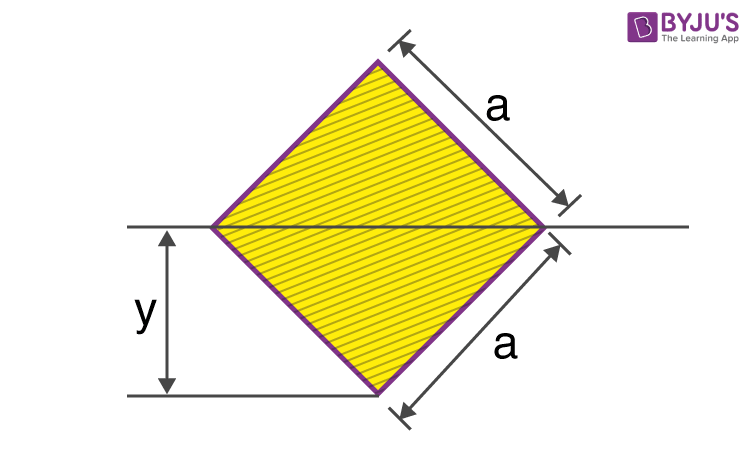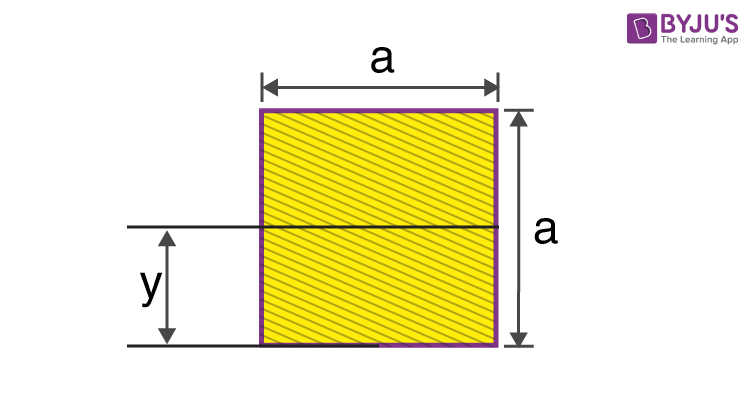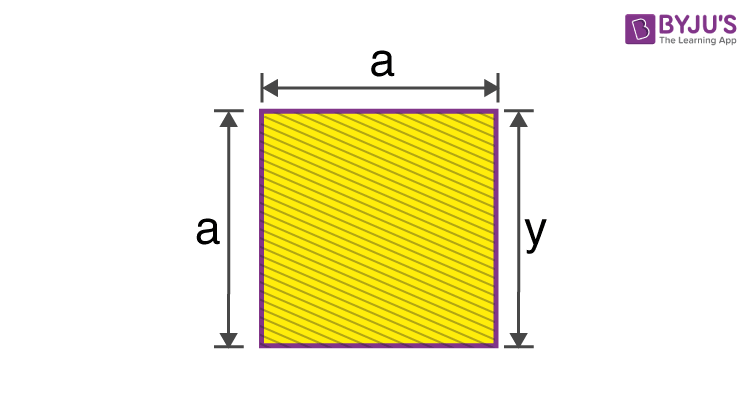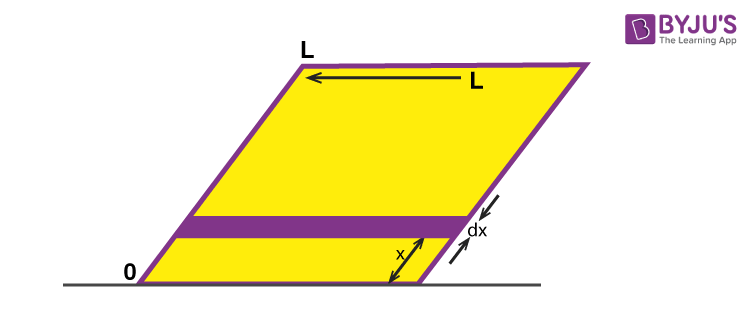Checkout JEE MAINS 2022 Question Paper Analysis : Checkout JEE MAINS 2022 Question Paper Analysis :

# Moment Of Inertia Of A Square

The moment of inertia of a square is given by the formula.

 I = a4 / 12

Here, a = sides of the square section. This equation is for a solid square where its centre of mass is along the x-axis.

The diagonal moment of inertia of a square can also be calculated as;

 Ix = Iy = a4 / 12Alternatively, if the centre of mass (cm) is moved to a certain distance (d) from the x-axis we will use a different expression for determining the moment of inertia of the same square.

 I = a4 / 3

## Moment Of Inertia Of A Square Derivation

The moment of inertia can be easily derived by using the parallel axis theorem which states;

cm = centre of mass

However, in this lesson, we will be replacing the mass (M) by area (A). For the derivation, we will also use a rectangle as a reference to find the M.O.I. along with integration.

If we recall the moment of inertia of a rectangle, it is given as;

IX = ⅓ WH3

W = width and H = height

IX = ⅓ (WH)H2

IX = ⅓ (A)H2

1. Now if we look at the square where its centre of mass passes through the x-axis, the square consists of two rectangles that are equal in size.We can now express it as;

Ix = 2 [⅓ a (a / 2)3 ]

Ix = [⅔ a ( a3 / 8) ]

Ix = (1/12)a4

IXcm = a4 / 12

2. The next derivation will be for a square when the centre of mass is moved to a certain distance (d).Using the parallel axis theorem we can now state;

Ix = (1/12) a4 + a2 (a / 2)2

Ix = (1/12) a4 + (1 / 4) a4

Ix = (1/12) a4 + (3 / 12) a4

Ix = (⅓) a4

## Moment Of Inertia of A Square PlateTo determine the moment of inertia of a square plate we have to consider a few things.

First, we will assume that the plate has mass (M) and sides of length (L). The surface area A = L X L = L2

Now we will define the mass per unit area as;

Surface density, ρ = M / A = M / L2

Using integration;

Iplate = ∫ dI = ∫ (dIcom + dIparallel axis)

Iplate = x=-L/2x=L/2 (1/12) ρ L3dx + ρ Lx2dx

Iplate = ρ (L3 / 12) [x |-L/2L/2 + ρ L [ ⅓ x3 |-L/2L/2

Iplate = ρ (L3 / 12) [ L / 2 – (-L / 2)] + ρ L [(⅓ L3 / 8) – (- ⅓ L3 / 8)]

Iplate = ρ (L3 / 12) (L) + ρ L (⅔ L3 / 8)

Iplate = (ρ / 12) L4 + (ρ / 12) L4

Iplate = (1 / 6) ρ L4

Iplate = (1 / 6) (M / L2) L4

Iplate = (1 / 6) M L2

## Parallel Axis Theorem